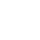CurrentHome>Resources
What is an FFT spectrum analyzer?
Release time :2021.04.21 Reading quantity:80

There are two techniques to accomplish signal frequency domain measurements (collectively referred to as spectrum analysis).

1. The FFT spectrum analyzer processes numerically the signal over a period of time and provides frequency, amplitude, and phase information. This instrument can also analyze periodic and non-periodic signals. FFT spectrum analyzer is characterized by fast speed and high accuracy. The frequency bandwidth is limited by the ADC sampling rate. So FFT spectrum analyzer is suitable for analyzing narrow bandwidth signals.

2. The Sweep spectrum analyzer is used to analyze stable and periodic signals and provide signal amplitude and frequency information. This type spectrum analyzer is suitable for fast, broadband tests.

In this artical, we will introduce some basic knowledge about FFT spectrum analyzer, and sweep spectrum analzyer in another artical.

Overview

FFT is short for Fast Fourier Transform. Fast Fourier Transforms is to calculate the frequency spectrum of the time domain signal. The signal must be digitized in the time domain and then an FFT algorithm is performed to get the spectrum.

Structure

1.  Input signal first passes through a variable attenuator to provide a proper amplitude range
2.  The signal passes through a low-pass filter to remove unwanted high-frequency components out of the instrument’s frequency range.
3.  Waveform sampling is analog-to-digital conversion, converted to digital form
4.  Data is saved into RAM
5.  The microprocessor (or other digital circuits such as FPGA, DSP) to receive the sample waveform, then calculate with FFT algorism to get the spectrum
6.  Display the result on the screen.

Advantage of FFT Spectrum Analyzer

FFT spectrum analyzers perform the same multichannel filter-like functions without many band-pass filters. The FFT spectrum analyzer uses digital signal processing to achieve the equivalent function of multiple independent filters. Conceptually, the FFT method is quite straightforward: digitize the signal and calculate the spectrum.

Still many factors need to be considered in order to do a meaningful measurement.

Limit of FFT Spectrum Analzyer

The essence of FFT is the baseband transform, in other words, the frequency range of FFT always starts from 0Hz and extends to some highest frequency. This would be a significant limitation to analyze a narrower frequency band signal (not start from DC).

How to Improve?

One way to increase frequency resolution is to increase the number of sample points N, which also increases FFT output points. However, it will also increase the size of the array FFT needs to process, and the result is increasing the calculating time. The computational time of the FFT algorithm often limits the performance of the instrument (such as screen refresh rate), so increasing the length of the FFT is often desirable.

Another approach is to use a digital downconverter to digitally down-convert a band-limited signal, which effectively reduces the sampling rate and improves frequency resolution. The structure is shown below:

1.  The output of the ADC is multiplied by the digital sine wave. Use digital mixer to down convert the digital sine wave
2.  The digital filter is set a proper filter factor to get appropriate frequency spacing. This bandwidth can be narrow enough to create frequency spacing as narrow as 1 Hz. Means the frequency resolution can achieve 1Hz.